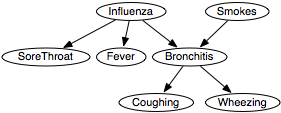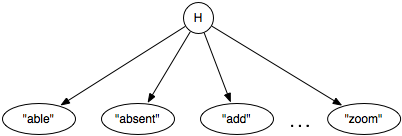### 6.3.1 Constructing Belief Networks

To represent a domain in a belief network, the designer of a network must consider the following questions:

• What are the relevant variables? In particular, the designer must consider
• what the agent may observe in the domain. Each feature that can be observed should be a variable, because the agent must be able to condition on all of its observations.
• what information the agent is interested in knowing the probability of, given the observations. Each of these features should be made into a variable that can be queried.
• other hidden variables or latent variables that will not be observed or queried but that make the model simpler. These variables either account for dependencies or reduce the size of the specification of the conditional probabilities.
• What values should these variables take? This involves considering the level of detail at which the agent should reason to answer the sorts of queries that will be encountered.

For each variable, the designer should specify what it means to take each value in its domain. What must be true in the world for a variable to have a particular value should satisfy the clarity principle. It is a good idea to explicitly document the meaning of all variables and their possible values. The only time the designer may not want to do this is when a hidden variable exists whose values the agent will want to learn from data (see Section 11.2.2).

• What is the relationship between the variables? This should be expressed in terms of local influence and be modeled using the parent relation.
• How does the distribution of a variable depend on the variables that locally influence it (its parents)? This is expressed in terms of the conditional probability distributions.
Example 6.14: Suppose you want the diagnostic assistant to be able to reason about the possible causes of a patient's wheezing and coughing, as in Example 5.30.
• The agent can observe coughing, wheezing, and fever and can ask whether the patient smokes. There are thus variables for these.
• The agent may want to know about other symptoms of the patient and the prognosis of various possible treatments; if so, these should also be variables. (Although they are not used in this example).
• There are variables that are useful to predict the outcomes of patients. The medical community has named many of these and characterized their symptoms. Here we will use the variables Bronchitis and Influenza.
• Now consider what the variables directly depend on. Whether patients wheeze depends on whether they have bronchitis. Whether they cough depends on on whether they have bronchitis. Whether patients have bronchitis depends on whether they have influenza and whether they smoke. Whether they have fever depends on whether they have influenza. Figure 6.3 depicts these dependencies.Figure 6.3: Belief network for Example 6.14

• Choosing the values for the variables involves considering the level of detail at which to reason. You could encode the severity of each of the diseases and symptoms as values for the variables. You could, for example, use the values severe, moderate, mild, or absent for the Wheezing variable. You could even model the disease at a lower level of abstraction, for example, by representing all subtypes of the diseases. For ease of exposition, we will model the domain at a very abstract level, only considering the presence or absence of symptoms and diseases. Each of the variables will be Boolean, with domain {true,false}, representing the presence or absence of the associated disease or symptom.
• You assess how each variable depends on its parents, which is done by specifying the conditional probabilities of each variable given its parents:
P(influenza)= 0.05
P(smokes)= 0.2
P(soreThroat|influenza)= 0.3
P(soreThroat|¬influenza)= 0.001
P(fever|influenza)= 0.9
P(fever|¬influenza)= 0.05
P(bronchitis|influenza∧smokes)= 0.99
P(bronchitis|influenza∧¬smokes)= 0.9
P(bronchitis|¬influenza∧smokes)= 0.7
P(bronchitis|¬influenza∧¬smokes)= 0.0001
P(coughing|bronchitis)= 0.8
P(coughing|¬bronchitis)= 0.07
P(wheezing|bronchitis)= 0.6
P(wheezing|¬bronchitis)= 0.001

The process of diagnosis is carried out by conditioning on the observed symptoms and deriving posterior probabilities of the faults or diseases.

This example also illustrates another example of explaining away and the preference for simpler diagnoses over complex ones.

Before any observations, we can compute (see the next section), to a few significant digits, P(smokes)=0.2, P(influenza)= 0.05, and P(bronchitis)=0.18. Once wheezing is observed, all three become more likely: P(smokes|wheezing)=0.79, P(influenza|wheezing)= 0.25, and P(bronchitis|wheezing)=0.992.

Suppose wheezing∧fever is observed: P(smokes|wheezing∧fever)=0.32, P(influenza|wheezing∧fever)=0.86, and P(bronchitis|wheezing∧fever)=0.998. Notice how, as in Example 5.30, when fever is observed, influenza is indicated, and so smokes is explained away.

Example 6.15: Consider the belief network depicted in Figure 6.2. Note the independence assumption embedded in this model: The DAG specifies that the lights, switches, and circuit breakers break independently. To model dependencies among how the switches break, you can add more arcs and perhaps more nodes. For example, if lights do not break independently because they come from the same batch, you can add an extra node that conveys the dependency. You would add a node that represents whether the lights come from a good or bad batch, which is made a parent of L1_st and L2_st. The lights can now break dependently. When you have evidence that one light is broken, the probability that the batch is bad may increase and thus make it more likely that the other light is bad. If you are not sure whether the lights are indeed from the same batch, you can add a node representing this, too. The important point is that the belief network provides a specification of independence that lets us model dependencies in a natural and direct manner.

The model implies that no possibility exists of there being shorts in the wires or that the house is wired differently from the diagram. In particular, it implies that w0 cannot be shorted to w4 so that wire w0 can get power from wire w4. You could add extra dependencies that let each possible short be modeled. An alternative is to add an extra node that indicates that the model is appropriate. Arcs from this node would lead to each variable representing power in a wire and to each light. When the model is appropriate, you can use the probabilities of Example 6.11. When the model is inappropriate, you can, for example, specify that each wire and light works at random. When there are weird observations that do not fit in with the original model - they are impossible or extremely unlikely given the model - the probability that the model is inappropriate will increase.

Example 6.16: Suppose we want to develop a help system to determine what help page a user is interested in based on the keywords they give in a query to a help system.

The system will observe the words that the user gives. Suppose that we do not want to model the sentence structure, but assume that the set of words will be sufficient to determine the help page. The user can give multiple words. One way to represent this is to have a Boolean variable for each word. Thus, there will be nodes labeled "able", "absent", "add",..., "zoom" that have the value true when the user uses that word in a query and false when the user does not use that word.

We are interested in which help page the user wants. Suppose that the user is interested in one and only one help page. Thus, it seems reasonable to have a node H with domain the set of all help pages, {h1,...,hk}.

One way this can be represented is as a naive Bayesian classifier. A naive Bayesian classifier is a belief network that has a single node - the class - that directly influences the other variables, and the other variables are independent given the class. Figure 6.4 shows a naive Bayesian classifier for the help system where H, the help page the user is interested in, is the class, and the other nodes represent the words used in the query. In this network, the words used in a query depend on the help page the user is interested in, and the words are conditionally independent of each other given the help page.Figure 6.4: Naive belief network for Example 6.16

This network requires P(hi) for each help page hi, which specifies how likely it is that a user would want this help page given no information. This information could be obtained from how likely it is that users have particular problems they need help with. This network assumes the user is interested in exactly one help page, and so i P(hi)=1.

The network also requires, for each word wj and for each help page hi, the probability P(wj|hi). These may seem more difficult to acquire but there are a few heuristics we can use. The average of these values should be the average number of words in a query divided by the total number of words. We would expect words that appear in the help page to be more likely to be used when asking for that help page than words not in the help page. There may also be keywords associated with the page that may be more likely to be used. There may also be some words that are just used more, independently of the help page the user is interested in. Example 7.13 shows how the probabilities of this network can be learned from experience.

To condition on the set of words in a query, the words that appear in the query are observed to be true and the words that are not in the query are observed to be false. For example, if the help text was "the zoom is absent", the words "the", "zoom", "is", and "absent" would be observed to be true, and the other words would observed to be false. The posterior for H can then be computed and the most likely few help topics can be shown to the user.

Some words, such as "the" and "is", may not be useful in that they have the same conditional probability for each help topic and so, perhaps, would be omitted from the model. Some words that may not be expected in a query could also be omitted from the model.

Note that the conditioning was also on the words that were not in the query. For example, if page h73 was about printing problems, we may expect that everyone who wanted page h73 would use the word "print". The non-existence of the word "print" in a query is strong evidence that the user did not want page h73.

The independence of the words given the help page is a very strong assumption. It probably does not apply to words like "not", where what "not" is associated with is very important. If people are asking sentences, the words would not be conditionally independent of each other given the help they need, because the probability of a word depends on its context in the sentence. There may even be words that are complementary, in which case you would expect users to use one and not the other (e.g., "type" and "write") and words you would expect to be used together (e.g., "go" and "to"); both of these cases violate the independence assumption. It is an empirical question as to how much violating the assumptions hurts the usefulness of the system.